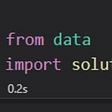# Levenshtein Distance for Dummies

Recently, I studied NLP to improve my knowledge in Computer Science. I read about it step by step and make me stuck in a thing called Levenshtein Distance. I know what is Levenshtein Distance about, but I don’t see how it works. Now, after many tutorials enlightened me, I will try to write it in human words.

First of all, I will give you the definition and application of Levenshtein Distance.

Levenshtein distance is a string metric for measuring the difference between two sequences. Informally, the Levenshtein distance between two words is the minimum number of single-character edits (insertions, deletions or substitutions) required to change one word into the other (wikipedia)

Levenshtein distance is named after the Russian scientist Vladimir Levenshtein, who devised the algorithm in 1965. If you can’t spell or pronounce Levenshtein, the metric is also sometimes called edit distance.

The Levenshtein distance algorithm has been used in:

Spell checking
Speech recognition
DNA analysis
Plagiarism detection

How does Levenshtein Distance work?

In a simple case, we can count the minimum character edits in two words. Like on the above Wikipedia explanation, edits are defined by either insertions, deletions or substitutions on one or more characters.

example:

1. Levenshtein Distance between FORM and FORK is 1. There is one substitution from M to K.

2. Levenstein Distance between KITTEN and SITTING is 3 because there are 3 characters edits.

## Levenshtein Distance Equation

Now we will use a dynamic programming approach to count the Levenshtein Distance between two words. We will use the above equation to compute the distance.

where
a = word a
b = word b
a and b are strings or words which we want to count the distance.
i and j is a matrix coordinate that helps us to count the edit.
In the last row of the equation, the +1 (plus one) operator only added when i and j are not the same character.

Here is the step to count the distance.

First of all, make a matrix which contains values like this.

And then we will count Lev(1,1) which is highlighted with a yellow box.

1. because of min(1,1)≠0, then we find the minimum of three equation
2. lev(0,1) means we find the value from row with index 0 and column with index 1
3. lev(1,0) means we find the value from row with index 1 and column with index 0
4. lev(0,0) means we find the value from row with index 0 and column with index 0. Because K is not equal with S, so we add plus one in the equation.
5. The value of min(2,2,1) is 1, so we can place 1 in the matrix above.

Now, we will find Lev(2,1), which is highlighted with a green box.

1. lev(1,1) means we find the value from row with index 1and column with index 1
2. lev(2,0) means we find the value from row with index 2 and column with index 0
3. lev(1,0) means we find the value from row with index 1and column with index 0. Because I is not equal with S, so we add plus one in the equation.
4. The value of min(2,3,2) is 2, so we can place 2 in the matrix above.

The following is a complete matrix if all steps are taken

The final Levenshtein Distance Value is always in the corner which is highlighted with a red box.

Thank you!

--

--

--

## More from Analytics Vidhya

Analytics Vidhya is a community of Analytics and Data Science professionals. We are building the next-gen data science ecosystem https://www.analyticsvidhya.com## Dea Venditama

Freelance Programmer & Fungsional Pranata Komputer Badan Pusat Statistik RI

## Using domain specific data to build better data processes## Build a Book Recommendation Bot with TigerGraph## Transfer Learning: Summarizing Medical Documents## Learn with Memgraph - Graph Modelling Course: Lesson #1